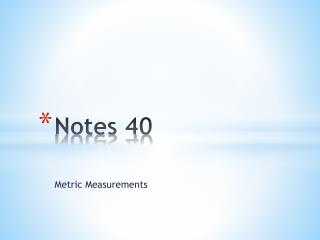DownloadDownload PresentationNotes 40

# Notes 40

Télécharger la présentation## Notes 40

- - - - - - - - - - - - - - - - - - - - - - - - - - - E N D - - - - - - - - - - - - - - - - - - - - - - - - - - -
##### Presentation Transcript

1. Notes 40 Metric Measurements

2. Additional Example 1: Choosing the Appropriate Metric Unit Choose the most appropriate metric unit for each measurement. Justify your answer. A. The amount of water a runner drinks eachday Liters—The amount of water a runner drinks each day is similar to the amount of water in a large water bottle. B. The length of a boat Meters—The length of a boat is similar to the length of several doorways. C. The mass of a car Kilograms—The mass of a car is similar to the mass of several hundred textbooks.

3. Check It Out: Example 1 Choose the most appropriate metric unit for each measurement. Justify your answer. A. The amount of liquid in 10 teardrops B. The mass of a pencil eraser C. The length of 15 soccer fields

4. Reading Math Prefixes: Milli- means “thousandth” Centi- means “hundredth” Kilo- means “thousand” To convert metric units, multiply or divide by a power of 10. Multiply to convert to a smaller unit and divide to convert to a larger unit.

5. Additional Example 2A: Converting Metric Units Convert the measure. 530 cL to liters 100 cL = 1L, so divide by 100. 530 cL = (530 ÷ 100) L Move the decimal point 2 places left: 530. = 5.3 L

6. Additional Example 2B: Converting Metric Units Convert the measure. 1,070 g to milligrams 1 g = 1000 mg, so multiply by 1000. 1,070 g = (1070  1000) mg Move the decimal point 3 places right: 1,070,000. = 1,070,000 mg

7. Check It Out: Example 2A Convert the measure. 980 dm to meters

8. Check It Out: Example 2B Convert the measure. 580 g to centigrams

9. Additional Example 3: Using Unit Conversion t to Make ComparisonsElizabeth purchases one pumpkin that weighs 3 kg and another that weighs 2,150 g. Which pumpkin weighs more? Use estimation to explain why your answer makes sense. You can convert the mass of Elizabeth’s pumpkin to grams. 1 kg = 1000 g, so multiply by 1,000. 3 kg = (3  1,000) g Move the decimal point 3 places right: 3.000. = 3,000 g 2,150 g is about 2 kg. Since 2 kg < 3 kg, Elizabeth’s 3 kg pumpkin weighs more.

10. Check It Out: Additional Example 3 Tyesha purchases a bag of potatoes that weighs 2.5 kg and another bag that weighs 3,850 g. Which bag weighs more? Use estimation to explain why your answer makes sense.